voltage is 0 when the input frequency is equal to the center frequency. When the input frequency rises above the center frequency, the output voltage goes positive.">Custom SearchGATED-BEAM DETECTOR An fm demodulator employing a completely different detection principle is the GATED-BEAM DETECTOR (sometimes referred to as the QUADRATURE DETECTOR). A simplified diagram of a gated-beam detector is shown in figure 3-16. It uses a gated-beam tube to limit, detect, and amplify the received fm signal. The output voltage is 0 when the input frequency is equal to the center frequency. When the input frequency rises above the center frequency, the output voltage goes positive. When the input frequency drops below the center frequency, the output voltage goes negative. Figure 3-16. - Gated-beam detector.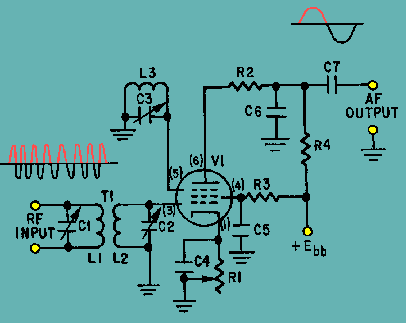Circuit Operation The gated-beam detector employs a specially designed gated-beam tube. The elements of this tube are shown in figure 3-17. The focus electrode forms a shield around the tube cathode except for a narrow slot through which the electron beam flows. The beam of electrons flows toward the limiter grid which acts like a gate. When the gate is open, the electron beam flows through to the next grid. When closed, the gate completely stops the beam. Figure 3-17. - Gated-beam tube physical layout.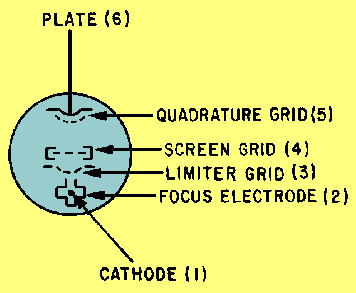After the electron beam passes the limiter grid, the screen grid refocuses the beam toward the quadrature grid. The quadrature grid acts much the same as the limiter grid; it either opens or closes the passage for electrons. These two grids act similar to an AND gate in digital devices; both gates must be open for the passage of electrons to the plate. Either grid can cut off plate current. AND gates were presented in NEETS, Module 13, Introduction to Number Systems, Boolean Algebra, and Logic Circuits. Look again at the circuit in figure 3-16. With no signal applied to the limiter grid (3), the tube conducts. The electron beam moving near the quadrature grid (5) induces a current into the grid which develops a voltage across the high-Q tank circuit (L3 and C3). C3 charges until it becomes sufficiently negative to cut off the current flow. L3 tends to keep the current moving and, as its field collapses, discharges C3. When C3 discharges sufficiently, the quadrature grid becomes positive, grid current flows, and the cycle repeats itself. This tank circuit (L3 and C3) is tuned to the center frequency of the received fm signal so that it will oscillate at that frequency. The waveforms for the circuit are shown in figure 3-18. View (A) is the fm input signal. The limiter-grid gate action creates a wave shape like view (B) because the tube is either cut off or saturated very quickly by the input wave. Note that this is a square wave and is the current waveform passing the limiter grid. Figure 3-18A. - Gated-beam detector waveforms.Figure 3-18B. - Gated-beam detector waveforms.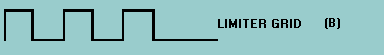At the quadrature grid the voltage across C3 lags the current which produces it [view (C)]. The result is a series of pulses, shown in view (D), appearing on the quadrature grid at the center frequency, but lagging the limiter-grid voltage by 90 degrees. Because the quadrature grid has the same conduction and cutoff levels as the limiter grid, the resultant current waveform will be transformed into a square wave. Figure 3-18C. - Gated-beam detector waveforms.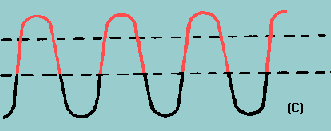Figure 3-18D. - Gated-beam detector waveforms.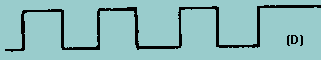Both the limiter and quadrature grids must be positive at the same time to have plate current. You can see how much conduction time occurs for each cycle of the input by overlaying the current waveforms in views (B) and (D), as shown in view (E). The times when both grids are positive are shown by the shaded area of view (E). These plate current pulses are shown for operation at resonance in view (F). Figure 3-18E. - Gated-beam detector waveforms.Figure 3-18F. - Gated-beam detector waveforms.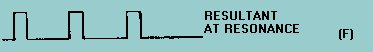Now consider what happens with a deviation in frequency at the input. If the frequency increases, the frequency across the quadrature tank also increases. Above resonance, the tank appears capacitive to the induced current; voltage then lags the applied voltage by more than 90 degrees, as shown in view (G). Note in view (H) that the two grid signals have moved more out of phase and the average plate current level has decreased. Figure 3-18G. - Gated-beam detector waveforms.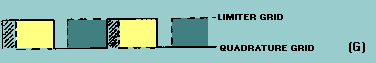Figure 3-18H. - Gated-beam detector waveforms.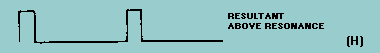As the input frequency decreases, the opposite action takes place. The two grid signals move more in phase, as shown in view (I), and the average plate current increases, as shown in view (J). Figure 3-18I. - Gated-beam detector waveforms.Figure 3-18J. - Gated-beam detector waveforms.View (K) shows the resultant plate-current pulses when an fm signal is applied to a gated-beam detector. Plate load resistor R4 and capacitor C6 form an integrating network which filters these pulses to form the sine-wave output. Figure 3-18K. - Gated-beam detector waveforms.Advantages of the Gated-Beam Detector The primary advantage of the gated-beam detector lies in its extreme simplicity. It employs only one tube, yet provides a very effective limiter with linear detection. It requires relatively few components and is very easily adjusted. There are more than the three types of fm demodulators presented in this chapter. However, these are representative of the types with which you will be working. The principles involved in their operation are similar to the other types. You will now briefly study PHASE DEMODULATION which uses the same basic circuitry as fm demodulators. Q.28 What circuit functions does the tube in a gated-beam detector serve?Q.29 What condition must exist on both the limiter and quadrature grids for current to flow in a gated-beam detector?Q.30 Name two advantages of the gated-beam detector.Integrated Publishing, Inc. - A (SDVOSB) Service Disabled Veteran Owned Small Business